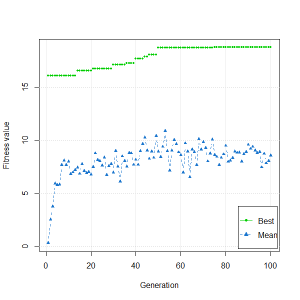Guest post by Kim Man Lui, PhD. (about GA – an R package for optimization using genetic algorithms)

The GA package (version 2.0) is a generic package in which one can define his/her own functions of fitness, selection, crossover and mutation. Among those functions, the most important is the fitness. However, in the GA package, the fitness function has been assumed to be deterministic. When we are dealing with a stochastic fitness function in financial computing, the GA package may provide a biased result. However, we may easily extend the package to deal with a stochastic fitness function.

Let us look at the following R code in which our fitness function is stochastic

f <- function(x) x*runif(x)

fitness <- function(x) f(x)

GA <- ga(type="real-valued", fitness=fitness, min=-20, max=20)

plot(GA)Fig 1: fitness <- function(x) x*runif(x)

Interestingly, if we take out the random (i.e. f <- function(x) x ) and there is not much difference in plot(GA) (see Fig 2) as the best always converges steadily.

Fig 2: fitness <- function(x) x

The result (Fig 1) is biased as the fitness of elite solution(s) in the next population is not re-calculated owing to the assumption of a deterministic fitness function. When coping with a stochastic process, we can do the following.

Type ga and save the source code. Look for the following lines and always set Fitness[]=NA as

for (iter in 1:maxiter) {

Fitness[]=NA #add this line to deal with a stochastic fitness function

if (!parallel) {

for (i in 1:popSize) if (is.na(Fitness[i])) {

Fitness[i] <- fitness(Pop[i, ], ...)

}

That is it! Now, re-run the code. plot(GA)will give a representative picture (see Fig 3) for the optimal solution in a stochastic process.

Fig 3: fitness <- function(x) x*runif(x) and elite solution(s) are re-calculated